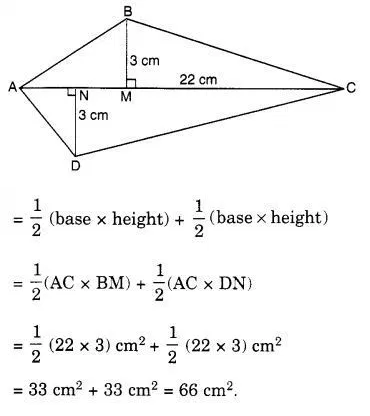# Find the area of the quadrilateral ABCD

Find the area of the quadrilateral ABCD.
Here, AC = 22 cm, BM = 3 cm, DN = 3 cm, and BM ⊥ AC, DN ⊥ AC.

Area of the quadrilateral ABCD = Area of the triangle ABC + Area of the triangle ADC